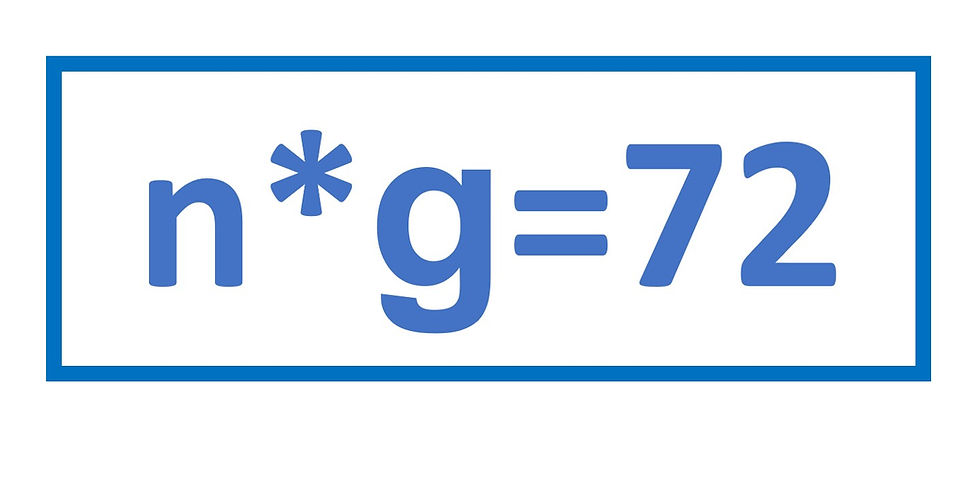top of page
Search
•John Krehbiel

# Rule of 72 Explained => Investment Math Nerd time!Here is a quick way to calculate how many years it will take for your investment to be worth twice as much. Take the number 72 and divide it by the growth rate or interest rate. For example, if you expect your investment to grow by 6% each year, it should take about 12 years (=72/6) to double in value. Another way to represent this is: (the number of years to double, n) multiplied by (the growth rate, g) equals 72. Algebraically, n*g=72.

Where does the Rule of 72 come from, mathematically?

If you hated math in school, you may want to stop now. The Rule of 72 is still useful, even if you don’t know where it comes from.

· If we start with an investment amount, P (for Principal) and it grows by a percentage, “g” (for growth), then

· The investment will have grown to P*(1+g) after one year.

· It will be worth P*(1+g)*(1+g) after 2 years.

· There are as many (1+g) terms as years, so after n (for number) years it will be worth

P*(1+g)^n.

We can use this to determine how many years it will take for P to double (i.e. become 2*P); we are trying to solve for n in the equation:

(a) P*(1+g)^n = 2*P

We can cancel the P on each side, so we get

(b) (1+g)^n = 2

To solve for an exponent, like n in this equation, we need to use logarithms. So we take the natural log of both sides:

(c) ln(1+g)^n = ln(2)

by the properties of logarithms and exponents, this becomes

(d) n*ln(1+g) = ln(2)

To manage ln(1+g) we will use a Taylor series expansion:

(e) ln(1+g) = g - (g^2)/2 + (g^3)/3 - (g^4)/4+ ……

Since g is a percentage, it is usually close to 0, so all the higher powers of g are very small compared to g (especially (g^3), (g^4), etc.). So let’s just use the first two terms, and approximate

(f) ln(1+g) = g - (g^2)/2

Substituting this into (d) above yields

(g) n*[g - (g^2)/2] = ln(2)

Multiplying the n through and calculating ln(2) = 0.693, yields

(h) n*g - n*(g^2)/2= 0.693

Adding the 2nd term on the left side to both sides gives

(i) n*g = 0.693 + n*(g^2)/2

We’ve isolated n*g on the left side of the equation (like in the first paragraph of this article), but the right side doesn’t equal 72 yet. First, g in the derivation above is a percentage equal to a small decimal (like 0.03 for 3%). So to get to the intentionally bold g in the n*g=72 equation, we need to multiply both sides by 100, because 100*g=g. Which gives:

(10) n*g= n*(100*g) = 100*[0.693+n*(g^2)/2]

Which simplifies to

(11) n*g= 69.3+100*n*(g^2)/2 = 69.3+50*n*g^2

So we have 69.3 on the right side, which is close to 72, along with an extra term, 50*n*g^2. It turns out that for the typical growth rates seen in investing (1% to 15%), the term 50*n*g^2 has a value between 0.4 and 5.0. This means n*g rounds off to a number between 70 and 74. 72 is right in the middle of that range. Also, very conveniently, 72 is evenly divisible by 2, 3, 4, 6, 8, 9, 12, 24, and 36.

For low growth rates (1%, for example), a “Rule of 70” would be more accurate, and for really high growth rates (100%, for example), this rule is not too useful, because at g=

100%, n*g=100, not 72.

Bottom Line:

The Rule of 72 (or n*g=72) is a good approximation to use to determine the amount of time it takes for an investment to double if you know the expected growth or interest rate.